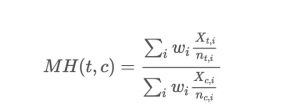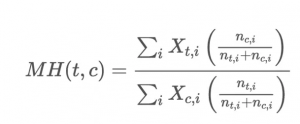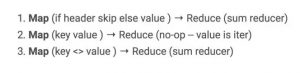## 数据科学的新生代工具(附实操代码)Mapreduce是一个简单平行数据处理的优秀工具，但如果想用Mapreduce处理更复杂的pipeline，问题出现了：

· 处理复杂pipeline需要关键的样本文件、独立的项目包 和层次之间的“界面”

· 在硬盘中写入pipeline层次之间的交互结果是一个瓶 颈，这要求使用者手动优化这些分支的设置

· 运行探究分析要求同时读写硬盘，这会变得很慢

· 不支持流pipeline（原始数据来源，极少延迟）

· 编写多层次pipeline时很容易被卡住，比如用曼特尔― 亨塞尔法（Mantel-Haenszel）衡量一个随机实验 参数和指标有效性（参见下文）

Spark和Beam/ Dataflow 通过将Mapreduce步骤的结果储存下来，优化和减少步骤，在内存中储存必要的交互结果，解决了以上的大部分困难。Dataflow中的PCollections和Spark中的RDD（resilient distributed dataset ）不需要写入硬盘，它们只需要重新存回内存里。

Beam对整个计算图进行详细读取的另一个影响是，Dataflow将作为一个功能存在。这种“将pipeline作为一种功能运行”的模式将读取计算图、输入数据，并输出数据。 Cloud Dataflow Service可以看成是一个黑匣子，处理的细节包括使用的虚拟存储器的数量、每一级甚至总体的层次、任务分配等等。需要的时候，这些信息都可以查看更多的细节。 这是Spark一个很显著的不同，因为Spark是聚类导向(集群导向， cluster-oriented)，而不是任务导向的。在Spark里运行一个固定pipeline时，我们会在建立集群cluster-运行pipeline-重建集群中循环。虽然有很多工具（比如 Cloud Dataproc on GCP 或 Databricks on AWS）简化这个过程，但用户依然非常重视集群管理。# We want to calculate MH(v_{t,i},n_{t,i},v_{c,i},n_{c,i}), where t and c are treatment

# and control. v and n in our cases are value of the sale prices and sale_count.

input_rdd = sc.textFile(‘sim_data_{0}_{1}.csv’.format(NUM_LE, NUM_HE))
header = input_rdd.first() # Remove the first line.
parsed_input_rdd = input_rdd.filter(lambda x: x !=header)

.map(lambda x: convert_line(x.split(‘,’)))
transformed = parsed_input_rdd.map(lambda x: ((x[exp], x[prod]),

(x[sale_count]*x

\$120/MONTH
, x[sale_count])))

(sp, clks) = (0, 1) # sale price and sale_count
(ep, spc) = (0, 1) # exp_id&product_id, sp&sale_count
(exp2, prod2) = (0, 1) # exp_id, product_id

# For each product cross exp_id, sum the sale prices and sale_count
grouped_result = transformed.reduceByKey(lambda x,y: (x[sp]+y[sp], x[clks]+y[clks]))
grouped_by_product = grouped_result.map(lambda x: ((x[ep][prod2]), (x[ep][exp2], x[spc][sp], x[spc][clks]))).groupByKey()

effect = numerator_sum / denominator_sum
print(numerator_sum, denominator_sum, effect)

Spark的功能有两种：transformations处理文档中（比如地图或文件夹）中记录的数据，actions让数据被识别（比如利用groupByKey， reduceByKey)。核心的区别就是是transformations只在覆盖到的地方执行，其他的部分不会产生直接的影响，这也是我们说Spark进行‘懒惰评估’

• sc.textFile(…) transforms a file on disk into an RDD (the distributed data structure in Spark)
• input_rdd.first() acts on the RDD returning first, header, element to the driver (my notebook).
• input_rdd.filter(…).map(…) transforms input_rdd removing the header then converts each csv line into floats and ints.
• parsed_input_rdd.map(…) transforms records into key-value tuples ((exp_id, product_id), (cost, clicks))
• transformed.reduceByKey(…) acts on transformed causing input_rdd.filter(…).map(…) and parsed_input_rdd.map(…) to be executed and produces the total clicks and cost by (exp_id, product_id)
• grouped_result.map(…).groupByKey() acts to produce the same data, only grouped by product_id instead of product_id and experiment_id.
• grouped_by_product.map(…).reduce(add) transforms the data per product_id into the numerator and denominator of the MH calculation and then performs the action of summing the results using the add function.

Dataflow跟Spark的的代码框架有少量的不同，总体上很类似，但却有很重要的区别。 区别中的一个缺点是，Dataflow至今还没有一个reduce的总功能，所以我要自己写代码(t_sum)。注意这个code用Dataflow SDK (而不是新的Beam SDK)。

Beam在概念上是两个部分：pipeline建设和pipeline执行。 beam.Pipeline（）返回一个pipeline，p，在其上构建（使用beam.Map，beam.GroupByKey等）和p.run（）在Dataflow的服务器或者在本地集群上执行Pipeline。

• beam.Pipeline(options=pipeline_options) begins constructing a pipeline to run locally.
• p | beam.io.Read(…) | beam.Filter(…) | beam.Map(…) add reading the file, filtering lines that look like the header (starting with ‘#’), converting each line into floats and ints to the graph.
• parsed_input_rdd | beam.Map(…) adds mapping each record to be keyed by exp_id, product_id to the graph
• transformed | beam.CombinePerKey(…) | beam.Map(…) | beam.GroupByKey() adds summing clicks and cost by exp_id, product_id and regrouping by product_id to the graph
• grouped_by_product | beam.Map(…) | beam.CombineGlobally(…) adds calculating the numerator/denominator values and the global sum to the graph
• numerator_sum | beam.Write(…) adds a sync for the numerator (there is a matching output for the denominator).
• p.run() optimizes constructed graph and ships the result to be executed (in our case the local machine)

Spark的优势：整合算法

# Load the data, remove the first line, and pick initial locations for the clusters.

# We put the x,y points in pt_rdd, and RDD of PtAgg (a class containing x, y,

# and count).
pt_rdd = parsed_input_rdd.map(lambda x: PtAgg(x, x, 1))
MAX_STEPS = 100; MIN_DELTA = 0.001; delta = 1.0; step = 0
while delta > MIN_DELTA and step < MAX_STEPS:
step += 1
c_centers_old = copy.deepcopy(c_centers)
# For every point, find the cluster its closer to and add to its total x, y, and count
totals = pt_rdd.map(lambda x: pick_closest_center(x, b_c_centers.value)).reduce(
lambda a,b: center_reduce(a,b))
# Now update the location of the centers as the mean of all of the points closest to it
# (unless there are none, in which case pick a new random spot).

c_centers = [t.Normalize() if t.cnt != 0 else random_point_location() for t in totals]

# compute the distance that each cluster center moves, the set the max of those as
# the delta used to the stop condition.

deltas = [math.sqrt(c.DistSqr(c_old)) for c, c_old in zip(c_centers, c_centers_old)]
delta = max(deltas)

s = ‘ ‘.join([str(x) for x in c_centers])
print(‘final centers: {0}’.format(s))

Beam/Dataflow的优势：流处理

1. 统一的批量和流API。

2. 支持处理实时数据的事件，也就是在它们发生的 时候就分析结果，而不是等到数据到达分析机器 的时候 。

3. 水印(一个追踪无界数据收集过程的方法)和窗口提 示完成进度(即收集完毕该阶段的所有数据)。

Dataflow通过”熔合”相同输入的同级层次来避免了caching。 熔合带来的局限性是不应该使用不稳定的类型，因为熔断的层次之间共享相同的数据，这样调和会产生无效的结果(可以通过run-time来核实)。 这意味着写正确的Beam代码需要对不稳定的类型格外注意。Spark默认双执行，比较慢但不需要担心正确的问题。Spark也支持不同类型的超高速缓冲方法。

Spark和Dataflow从不同方面解决了Mapreduce的不足，哪一个系统更适合得取决于项目本身。如果是交互性，数据探索或算法开发方面的，Spark可能是更好的选择。如果处理复杂的流计算（特别是实时事件），Beam可能会表现更好。而对于运行大规模生产pipeline，Beam on Cloud Dataflow也许更适合。